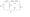# Time : 3 hours                                     Maximum Marks : 70

Attempt any five questions. Symbols and notations have their usual meaning.

Assume suitable missing data, if any

1. (a) Explain the following:

(i)         Permeability

(ii)       Hysteresis

(ii) Mutual Inductance.

(b) A 4-pole, long shunt, lap wound generator supplies 25 kW  at a terminal voltage of 500 V.

The armature resistance is 0*03 £2, series field resistance is 0*04 Q and shunt field resistance is 200 Q. The brush drop may be taken as 1 V. Determine the emf generated*         8

1. (a) Explain the following:

(i)       Difference between moving coil and moving iron instruments.

(ii)     Methods for extending the range of voltmeter.

{Hi) Gravity control system is usually not used in electrical measuring instruments. 3×3=9

(b) Obtain Thevenin’s equivalent circuit at A and B, as shown in Fig. 1.  51. (a) Derive the emf equation of a dc generator. For designing high voltage dc generator which type of winding should be used and why?                            7

(b) A dc shunt machine connected to 230 V supply ‘

has a resistance of armature as 0*115 £2 and of field winding as 115 Q. Find the ratio of the speed as a generator to the speed as a motor with the line current in each case being 100 A.

1. (a) Deduce the relationship between the phase and line quantities (voltages and currents) in three phase delta connected circuit.

Draw the phasor diagram to represent the above.

(b) In the circuit of Fig. 2 determine:

(i) The power supplied by the source

(«) The reactive power supplied by the source

(iii)     The reactive power of the capacitor

(iv)     The power factor of the circuit.                       8

1. (fl) Develop the equivalent circuit of a single phase transformer. Mention the physical significance of all its parameters.

(b) A 5 kVA single phase, 230/400 V two winding transformer is connected as an autotransformer for 230/630V. Find:

(t) load current and current in each winding at the full permissible output

(«) efficiency at full load, if iron loss and full load copper loss are 30 W and 75 W res­pectively.      8

1. (a) Derive the expression for developed torque in a

three phase induction motor and find the condition for maximum torque.      6

(b)     In a 6-pole, 3 phase, 50 Hz motor with star- connected rotor, the rotor resistance per phase is 0-3 Q, the leakage reactance at standstill is 1 *5 Q per phase, and an emf between the slip-rings on open circuit is 175 V.

Calculate:

(i) Slip at a speed of 950 rpm

(«) Rotor emf per phase

(Hi) Rotor frequency at a speed of 950 rpm

(iV) Rotor leakage reactance at a speed of 950 rpm.   8

1. (a) Derive emf equation of alternator and explain coil

span factor and distribution factor.                  7

(&) Explain the principle of operation of a synchronous motor and V-curve.        7

1. Write short notes on any two of the following:

(a)      Diversity Factor and Demand Factor

(b)      Megger

(c)      Tariffs

(d)      Armature Reaction.